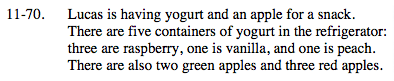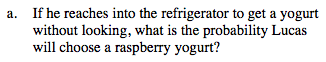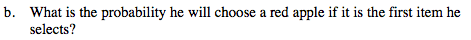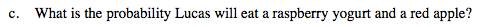### Home > MC2 > Chapter 11 > Lesson 11.2.4 > Problem11-70

11-70.How many raspberry yogurts are there out of the total number of yogurts in the refrigerator?How many red apples are there out of all the apples?Lucas' choices for the apple and yogurt are independent because the outcome for choosing one type of food will not affect his choice when choosing for the other type.

To find the probability of two independent events both occurring, find each probability separately and then multiply their probabilities.

$\frac{3}{5}\cdot\frac{3}{5}=\frac{9}{25}$

Use the eTool below to test the probability.
Click the link at right for the full version of the eTool: MC2 11-70 HW eTool